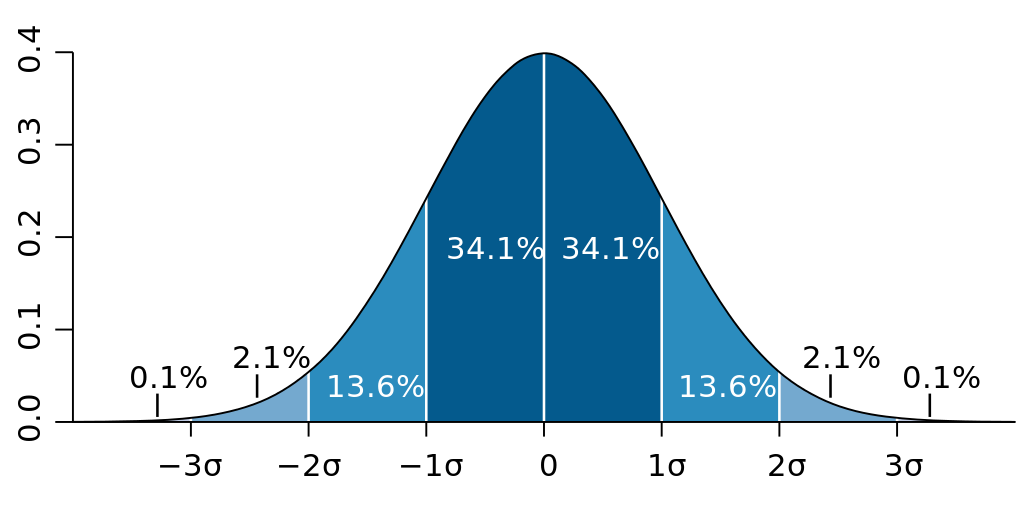# Standardize

Standard scaling of a FluidDataSet's contents

Standardized data means that every dimension in the data set has a mean of 0 and a standard deviation of 1. This scaling can be useful for many machine learning algorithms, but since most data in the wild does not fit this criteria, standardizing data is often employed. Standardizing data (and scaling data generally) can also be important for transforming a DataSet so that all of the dimensions have similar ranges. With similar ranges, the distance metrics (such as euclidian distance) used by many machine learning algorithms will similarly weight each of the dimensions when calculating how near or far (similar or dissimilar) two data points are from each other.

Using standardization to scale data implies the assumption that the data is generally normally distributed. Small data sets derived from audio analyses are often not normally distributed and therefore standardization might not be the best choice for scaling. It maybe useful to test other scalers (Normalize or RobustScale) and see which provides the best musical results.When data is normally distributed, it follows the 68/95/99.7 rule, indicating how much of the data is found within standard deviations of the mean. (image reproduced from Wikipedia)